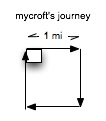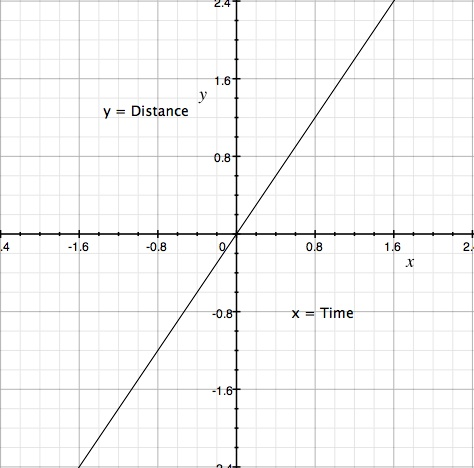Natural Science - Year I

#### Course Materials are always under revision! Weblecture content may change anytime prior to two weeks before scheduled chat session for content.Science Web Assignment for Unit 9

 This Unit's Homework Page History Lecture Science Lecture Lab Parents' Notes

# Science Lecture for Unit 9:Characteristics of Motion

##### For Class
• Topic area: Physics: Kinematics
• Terms and concepts to know: distance, displacement, speed, velocity, instantaneous change, average, acceleration
• See historical period(s): Explaining How Things Move

## Explaining How Things Move

The study of motion is called kinematics. We say something moves when it changes position, and goes from one place to another. So before we can even begin to discuss movement, we have to think about what we mean by place.

Think about how you give directions to your house. You may start with a nearby freeway ramp, or the downtown area, or, if you know where your audience lives, with their own home. When we give directions to where "here" is, we usually have to use some common reference point that everyone agrees on. We may even agree on some convention for directions, like using north, south, east, and west. In order to completely describe the difference in position between two points, we'll also need to agree on how we will measure the distance, that is, what kind of units we will use.

The combination of reference points, directions, and units make up our frame of reference. We'll use this concept a lot as we discuss motion in particular, and move from our everyday experience of it through the formal definitions of Isaac Newton's laws of motion, to the implications of Albert Einstein's theory of relativity. For now, though, we just want to understand what we mean by the motion in the most common sense, and learn how to measure and study it.

##### Distance Units

We commonly use two kinds of units to measure distance: the British system, which is based on measures historically used in the British Empire and America, and the Systeme International or SI system, which is based on the metric system. Each system has different units based on scale or overall size.. The foot, yard, and mile of the British system are used for small-scale, medium-scale, and large-scale distances. There are 12 inches in a foot, 3 feet in a yard, and 5280 feet or 1760 yards in a mile. The reason these have such odd relationships is that each unit was identified first, then the equivalents were determined as a kind of standard.

In the metric system, which is used by most scientists even in countries that still normally use the British system, each unit is related to the next by a power of ten. There are 100 centimeters in a meter, 1000 meters in a kilometer. This makes conversion between units designed for different scales easier.

When we measure distances, we chose units appropriate to the scale that we are measuring. For very small distances, such as that between the nucleus of a hydrogen atom and its electrons, we would use nanometers, or lengths 1/1 000 000 000th of a meter. For large distances, such as that to the sun or the next star, we use special astronomical measures such as the astronomical unit or light years or parsecs. As long as we are measuring distance, we could use any one of these units and convert from unit to unit without changing the laws of motion that govern the relationships of distance, speed, and acceleration.

#### Distance and DisplacementNow that we have some units, let measure some distances. Suppose that mycroft decides to take a walk. He leaves home, goes out a west-facing door, turns right, walks a mile, turns right, walks a mile, turns a third time, walks a mile, and turns again. After walking a final mile, how far is he from home?

Take a look at our little diagram. Our friendly bot is back in front of his own door. The distance he walked is four miles, but the displacement from his point of origin is zero. This distinction turns out to be important in modern physics: distance covered and distance from a reference point are not the same thing. We call the difference from a reference point displacement. It has both magnitude (how long a distance) and direction. When mycroft leaves his west-facing door and turns right, he is walk northward. At the end of his first mile, he is one mile north of home. At the end of his second mile, although he has walked two miles, he is about 1.414 miles to the north-east of home.

#### Speed and Velocity

Motion always involves a change of position in a given period of time. We can make a ratio of distance divided by time ("miles per hour", "furlongs per fortnight", "meters per second"), which we call speed. Any length/time calculation results in a "speed" value. If we add in the component of direction, speed becomes velocity. We can plot distance against time. Some of you may already have had some experience with such plots. We use a grid, and count up from our point of origin along the y-axis for distance. We count along the x-axis for time.We can map position as a function of time. Suppose that we look at mycroft's afternoon walk, where he simply started covering territory. We can make a table of mycroft's position at any given point in time.

 Position (y axis value) Time (x axis value) Speed (y/x) .6 .4 .6/.4 = 1.5 1.2 .8 1.2/.8 = 1.5 2.4 1.6 2.4/1.6 = 1.5

The speed is constant. If you've done any algebra, though, you know that what we've done is an analysis of the linear function y = f(x) = mx, where m is the slope of the line. Before you faint, that's just to say that the value we want to find (y) is a function of x, and the function is that we multiply m * x. For each x, there is one and only one value for y. But if y is distance and x is time, we can figure out what m is in this case. We can solve y = mx for m by dividing both sides of the equation by x. The x cancels on the right, and we have y/x = m. We already know that this means distance/time, so m is velocity. In other words, if we plot distance over time, the slope of the line at any point is the velocity of the moving object..

#### Acceleration

So far, we have a straight line. The slope = 1.5 at an point on the line, so the slope isn't changing, and the velocity isn't changing. But that isn't usually the case. Often we have a changing velocity. The rate or change of the velocity over time is called acceleration.

This isn't as difficult as it sounds. You've all heard advertisements for cars that claim the car can accelerate "from zero to sixty in 10.2 seconds". [This is an important consideration when you are trying to merge into rapidly moving traffic from a very short on-ramp.] The change from 0 miles per hour to 60 miles per hour in 10.2 seconds is acceleration.Let's see what happens when velocity changes over time. Suppose we shoot an arrow into the air. As it rises, the earth's gravitational pull decelerates it, slowing it down, then pulls it back down to earth. This time, we plot distance above the ground over time, taking as "zero time" the top of the arrow's flight. We won't worry about how the arrow moves along the surface of the earth; we are only concerned with its motion in one dimension, up/down. Notice that now we have a curved line. The slope is constantly changing. Since we already know that in a plot of distance against time, the slope means velocity, a curved slope must mean the velocity is changing, or undergoing acceleration.

How do we determine the acceleration at any given point in time? This was Aristotle's dilemma! He didn't have a very good clock, so it was difficult for him to determine how to plot position against the passage of time, even to investigate motion to the extent of figuring out where his arrow was. It is difficult enough for us to do, even with cameras and other devices, but one way is to approximate and find the average change over some small interval. If we look at our chart, we see that at 0 seconds (x = 0, v = 0), the position y is 10 meters (we have to have units for distance). At 1 second it is 5 meters from the origin. The velocity for this second of time is 5m/1s = 5 m/s. At the end of 2 seconds, the position is -5m (5 meters below the point of origin). The change in distance is 5m - (-5m) = 10m. The average velocity for this second second is total distance/time = 10m/1s = 10 m/s. The change in velocity from the 5m/s at the end of the first second to the 10 m/s at the end of the second second is 10m - 5m = 5 m/s in that second, or 5 meters/second/second, which physicists usually write (to everyone else's confusion) as 5 meters/second2.

We can have acceleration without changing speed. Remember that velocity is both speed and direction; acceleration is any change in velocity, so acceleration can be a change in either speed or direction or both. Think of a a marble rolling round and round in the bottom of a glass bowl with almost no friction. Its speed is constant, but its direction is constantly changing: it is going in a circle. Electrons moving around the nuclei of atoms and planets moving around the sun are similar examples of situations where speed varies little (if at all) but direction changes constantly. The electrons, the planets, and the marble are all accelerating, even though they are not "speeding up".

So we can make some definitions clearly now:

• Distance is a change in position. If we want to take direction into account, we use displacement as the term instead.
• Speed is the rate of change in position; velocity is speed in a particular direction.
• Acceleration is the rate of change in velocity.

We also know that if we graph y against x, the slope we get is the rate of change of y with respect to x. If x plots time, then we get the rate of change in time for y.

What happens if acceleration is changing? Well....things get very interesting. We can't use averages any more to determine acceleration. We have to use a mathematical technique called calculus, which wasn't available, at least in the western tradition, until Newton and Leibniz discovered how to do at about the same time in the seventeenth century.

##### Test yourself

mycroft goes jogging. He leaves the house at 9:10am. He is 1 mile from home at 9:20am. What is his speed in miles per hour?

mycroft manages to go only 1/2 of a mile in the next ten minutes. What is his average acceleration?

### Study/Discussion Questions

• What is the difference between distance and displacement? Why does it matter?
• What is the difference between average and instantaneous rates of change?
• What are some of the differences in determining rates of change?
• What are some of the problems involved in measuring velocity and acceleration?

### Further Study On your Own (Optional)

• The Velocity and Acceleration page at CK-12 has more information about velocity, distance, time, and how they are related. Be sure to play with the interactive simulator and note how the graphs display information about distance traveled over time and speed changes due to acceleration over time.